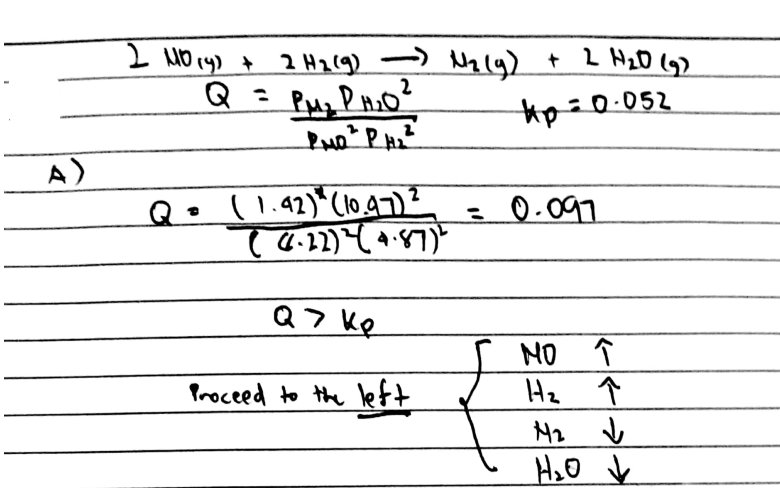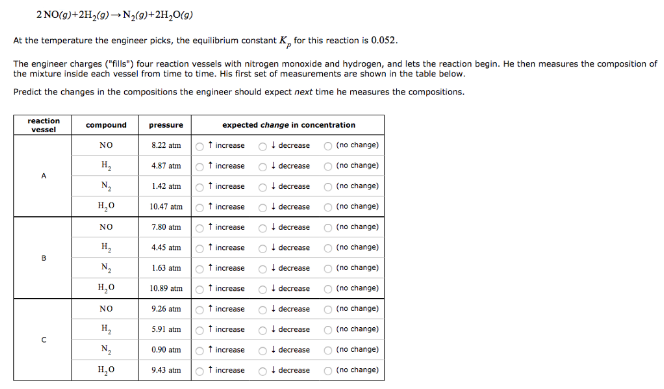# Problem: 2NO (g) + 2H2 (g) → N2 (g) + 2H2O (g) At the temperature the engineer picks, the equilibrium constant Kp for this reaction is 0.052. The engineer charges ("fills") four reaction vessels with nitrogen monoxide and hydrogen, and lets the reaction begin. He then measures the composition of the mixture inside each vessel from time to time. His first set of measurements are shown in the table below. Predict the changes in the compositions the engineer should expect next time he measures the compositions.

###### FREE Expert Solution83% (3 ratings)###### Problem Details

2NO (g) + 2H2 (g) → N2 (g) + 2H2O (g)

At the temperature the engineer picks, the equilibrium constant Kp for this reaction is 0.052.

The engineer charges ("fills") four reaction vessels with nitrogen monoxide and hydrogen, and lets the reaction begin. He then measures the composition of the mixture inside each vessel from time to time. His first set of measurements are shown in the table below.

Predict the changes in the compositions the engineer should expect next time he measures the compositions.